NCERT Solutions for Class 9 Maths Chapter 4 Lines and Angles Ex 4.2 are part of NCERT Solutions for Class 9 Maths. Here we have given NCERT Solutions for Class 9 Maths Chapter 4 Lines and Angles Ex 4.2.

 Board CBSE Textbook NCERT Class Class 9 Subject Maths Chapter Chapter 4 Chapter Name Lines and Angles Exercise Ex 4.2 Number of Questions Solved 6 Category NCERT Solutions

## NCERT Solutions for Class 9 Maths Chapter 4 Lines and Angles Ex 4.2

Question 1.
In figure, find the values of x and y and then show that AB || CD.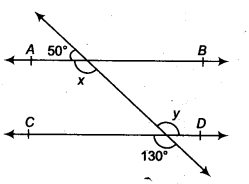Solution:
∵ x + 50° = 180° (Linear pair)
⇒ x = 130°
∴ y = 130° (Vertically opposite angle)
Here, ∠x = ∠COD = 130°
These are corresponding angles for lines AB and CD.
Hence, AB || CD

Question 2.
In figure, if AB || CD, CD || EF and y: z = 3:7, find x.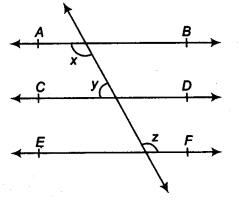Solution:
Given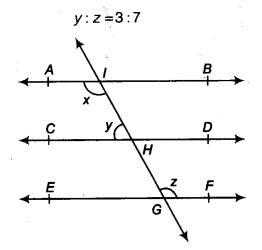⇒ Let y = 3k, z = 7k
x = ∠CHG (Corresponding angles)…(i)
∠CHG = z (Alternate angles)…(ii)
From Eqs. (i) and (ii), we get
x = z …….(iii)
Now, x+y = 180°
(Internal angles on the same side of the transversal)
⇒ z+y = 180° [From Eq. (iii)]
7k + 3k = 180°
⇒ 10k = 180°
⇒ k = 18
∴ y = 3 x 18° = 54°
and z = 7x 18°= 126°
∴ x = z
Now, x + y = 180°
(Internal angles on the same side of the transversal)
⇒ z + y = 180° [From Eq. (iii)]
7k + 3k = 180°
⇒ 10k = 180°
⇒ k = 18
∴ y = 3 x 18° = 54°
and z = 7x 18°= 126°
x = z
⇒ x = 126°

Question 3.
In figure, if AB || CD, EF ⊥ CD and ∠GED = 126°, find ∠AGE, ∠GEF and ∠FGE.Solution:
∵ ∠AGE = ∠GED (Alternate interior angles)
But ∠GED = 126°
⇒ ∠AGE = 126° ….(i)
∴ ∠GEF + ∠FED= 126°
⇒ ∠GEF + 90° =126° (∵ EF ⊥ CD)
⇒ ∠GEF = 36°
Also, ∠AGE + ∠FGE = 180° (Linear pair axiom)
⇒ 126° + ∠FGE =180°
⇒ ∠FGE = 54°

Question 4.
In figure, if PQ || ST, ∠ PQR = 110° and ∠ RST = 130°, find ∠QRS.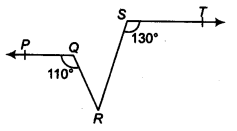Solution:
Drawing a tine parallel to ST through R.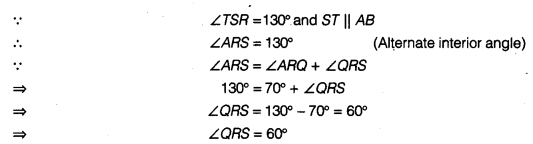Question 5.
In figure, if AB || CD, ∠APQ = 50° and ∠PRD = 127°, find x and y.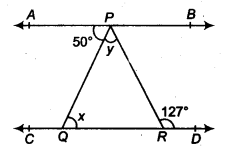Solution:
We have, AB || CD
⇒ ∠APQ = ∠PQR (Alternate interior angles)
⇒ 50° = x
⇒ x = 50°
Now, ∠PQR + ∠QPR = 127°
(Exterior angle is equal to sum of interior opposite angles of a triangle)
⇒ 50°+ ∠QPR = 127°
⇒ y = 77°.

Question 6.
In figure, PQ and RS are two mirrors placed parallel to each other. An incident ray AB strikes the mirror PQ at B, the reflected ray moves along the path BC and strikes the mirror RS at C and again reflects back along CD. Prove that AB || CD.Solution:We hope the NCERT Solutions for Class 9 Maths Chapter 4 Lines and Angles Ex 4.2 help you. If you have any query regarding NCERT Solutions for Class 9 Maths Chapter 4 Lines and Angles Ex 4.2, drop a comment below and we will get back to you at the earliest.